# Problem 57993. Easy Sequences 110: Integration of the Sum of a Recursive Trigonometric Function

A trigonometric function,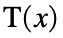, is defined as follows: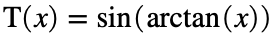, x in radians
Applyingrecursively we define another function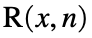, for integer n:We then define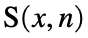as the sum of value of R from 1 to n:Finally, we are asked to evaluate the integral of S with respect to x, over the real range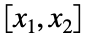:For example for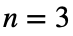,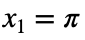,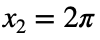, we have:
>> a = integral(@(x) sin(atan(x))+sin(atan(sin(atan(x))))+sin(atan(sin(atan(sin(atan(x)))))),pi,2*pi)
a = 7.05797686912156
Please present the final output rounded-off to 6 decimal places. Therefore the final answer is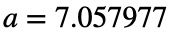.
-------------------------
NOTE: There are a number of ways to do numerical Integration in Matlab. Just make sure that the output would be accurate within 6 decimal places of the value obtained using the integral function shown above.

### Solution Stats

100.0% Correct | 0.0% Incorrect
Last Solution submitted on Jun 05, 2023

### Community Treasure Hunt

Find the treasures in MATLAB Central and discover how the community can help you!

Start Hunting!